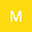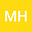Blow-up for wave equation with the scale-invariant damping and combined nonlinearities
••• Makram Hamouda,
• M.Ali Hamza
Makram Hamouda
Imam Abdulrahman Bin Faisal University

Corresponding Author:mahamoud@indiana.edu

Author ProfileM.Ali Hamza
Imam Abdulrahman Bin Faisal University
Author Profile## Abstract

In this article, we study the blow-up of the damped wave equation in the \textit{scale-invariant case} and in the presence of two nonlinearities. More precisely, we consider the following equation: \begin{displaymath} \d u_{tt}-\Delta u+\frac{\mu}{1+t}u_t=|u_t|^p+|u|^q, \quad \mbox{in}\ \R^N\times[0,\infty), \end{displaymath} with small initial data.\\ For $\mu < \frac{N(q-1)}{2}$ and $\mu \in (0, \mu_*)$, where $\mu_*>0$ is depending on the nonlinearties’ powers and the space dimension ($\mu_*$ satisfies $(q-1)\left((N+2\mu_*-1)p-2\right) = 4$), we prove that the wave equation, in this case, behaves like the one without dissipation ($\mu =0$). Our result completes the previous studies in the case where the dissipation is given by $\frac{\mu}{(1+t)^\beta}u_t; \ \beta >1$ (\cite{LT3}), where, contrary to what we obtain in the present work, the effect of the damping is not significant in the dynamics. Interestingly, in our case, the influence of the damping term $\frac{\mu}{1+t}u_t$ is important.
23 Jun 2020Submitted to Mathematical Methods in the Applied Sciences
27 Jun 2020Submission Checks Completed
27 Jun 2020Assigned to Editor
02 Jul 2020Reviewer(s) Assigned
30 Jul 2020Review(s) Completed, Editorial Evaluation Pending
31 Jul 2020Editorial Decision: Revise Minor